﻿ 文档嵌入维度同胚——政府工作报告实例分析 Document Embedding Dimension Homeomorphism—Government Work Report Analysis

Computer Science and Application
Vol. 10  No. 06 ( 2020 ), Article ID: 36075 , 15 pages
10.12677/CSA.2020.106124

Document Embedding Dimension Homeomorphism

—Government Work Report Analysis

Hualun Xie1, Xun Liang1, Mengdie Li2

1School of Information, Renmin University of China, Beijing

2Government Service Center of Kaifu District, Changsha Hunan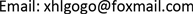Received: May 27th, 2020; accepted: Jun. 5th, 2020; published: Jun. 12th, 2020ABSTRACT

The intelligent analysis of big data in government work reports can quickly and fully grasp the correlation of various internal factors and support decision maker to complete reasonable judgment. Taking the 18-year government work report as research target, which consists of 31 provinces and municipalities at the county level and above after 2000, this paper first proposes the optimal embedding dimension analysis framework based on document embedding homeomorphic space. And then on this basis, the paper studies the separability and similarity of provincial documents of the best document embedded dimension homeomorphism model of government work report. Finally, the experimental results and analysis of the document clustering separability and similarity difference in the government work report are given. The optimal document embedding vector obtained by the model can effectively divide the provincial subspace of the government work report, and the differences in the similarity of the provincial time series of the local government work report highlight their gaps in politics, economy, education, culture and so on. The experiment also found that the Euclidean distance using the regularized government document vector is equivalent to the traditional cosine distance. The document embedding analysis framework not only has certain reference significance and application value for the construction of smart government affairs in China, but also can support deep information mining, report reinterpretation and intelligent decision making in multi-category documents such as public company announcements.

Keywords:Government Work Report, Document Embedding, Homeomorphism, Dimension——政府工作报告实例分析

1中国人民大学信息学院，北京

2湖南省长沙市开福区政务服务中心，湖南 长沙1. 引言

2. 政府文档嵌入维度分析

Bengio提出一个自然语言处理的大规模深度学习模型，这个模型能够通过学习单词的分布化表示来获得上下文信息 。基于神经网络的分布式表示一般称为词向量、词嵌入(word embedding)或分布式表示(distributed representation)，核心依然是上下文的表示以及上下文与目标词之间的关系的建模(见图1)。相比于词的独热表示，一是将词向量的每一个元素由整型改为浮点型，变为整个实数范围的表示，二是将原来稀疏的巨大维度压缩嵌入到一个更小维度的空间。

Milokov等人提出了Word2Vec词嵌入算法，Google将其于2013年开源，采用了CBOW (continuous bag-of-words model)和skip-gram (continuous skip-gram model)两种模型与负采样和层次损失函数两种方法 。随后的2014年，Le和Mikolov提出了文本向量的深度学习算法Doc2Vec文档嵌入(document embedding)，借鉴Word2Vec思想的两种结构：PV-DM和PV-DBOW，分别对应Word2Vec中的CBOW和skip-gram 。Doc2Vec在神经网络的输入层增加了一个唯一的文档(段落)ID，在计算句子向量的同时算法也计算出词向量(见图2)。在图2中，政府文档用矩阵D中一行唯一表示，词用one-hot词汇表W中一行唯一表示。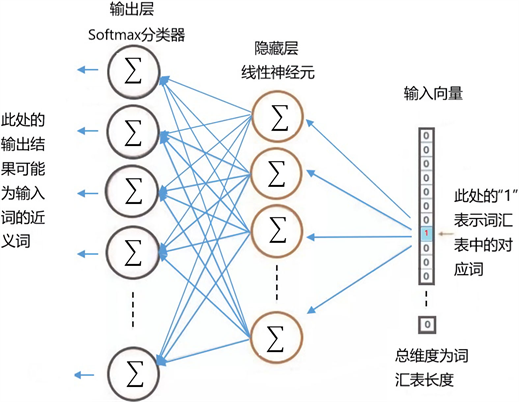Figure 1. Word vectors are hidden layer parameters when training neural networks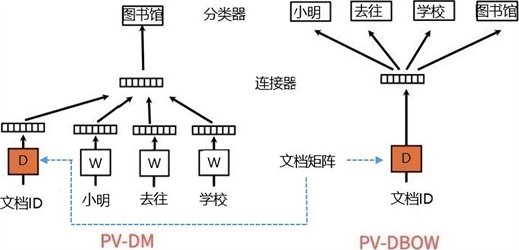Figure 2. Two structures of Doc2Vec

2.1. 文档嵌入的几何性质与几何不变性

· 相似性。文档的相似性可以通过向量夹角的余弦值来表示。对于两个文档向量Vi和Vj，它们夹角的余弦值 $\mathrm{cos}\left({V}_{i},{V}_{j}\right)$ 的范围在−1到1之间。这个值越大，代表第i个和第j个文档之间的相似度越高；

· 类比性。类比性考察的是两篇文档之间作者是否相同。文档类比这一语义性质对应的几何性质为两对文档向量的差的平行度；

· 聚类性。相似文档向量之间的距离总是较小，因而这些文档会倾向于聚成一类。

2.2. 政府文档嵌入的损失函数

$\text{DVM}\left(E\right)=E{E}^{\text{T}}$

$\text{DVML}\left({E}_{1},{E}_{2}\right)=‖\text{DVM}\left({E}_{1}\right)-\text{DVM}\left({E}_{2}\right)‖=‖{E}_{1}{E}_{1}^{\text{T}}-{E}_{2}{E}_{2}^{\text{T}}‖=\sqrt{\underset{i,j}{\sum }{\left(\left({v}_{i}^{\left(1\right)},{v}_{j}^{\left(1\right)}\right)-\left({v}_{i}^{\left(2\right)},{v}_{j}^{\left(2\right)}\right)\right)}^{2}}$

3. 政府文档嵌入流形学习分析

3.1. 高维空间政府文档嵌入

3.2. 政府文档向量距离

$\text{DCOS}\left(A,B\right)=\frac{{\sum }_{i=1}^{n}\left({A}_{i}{B}_{i}\right)}{‖A‖‖B‖}$

$\text{DEUC}\left(A,B\right)=\sqrt{{\sum }_{k=1}^{n}{\left({x}_{k}^{*}-{y}_{k}^{*}\right)}^{2}}$${x}_{k}^{*}=\frac{{x}_{k}}{\sqrt{{x}_{1}^{2}+{x}_{2}^{2}+\cdots +{x}_{n}^{2}}}$

$\begin{array}{c}\text{DEUC}\left(A,B\right)=\sqrt{{\sum }_{k=1}^{n}{\left({x}_{k}^{*}-{y}_{k}^{*}\right)}^{2}}\\ =\sqrt{{\sum }_{k=1}^{n}{\left({x}_{k}^{*}\right)}^{2}+{\sum }_{k=1}^{n}{\left({y}_{k}^{*}\right)}^{2}-2\left({\sum }_{k=1}^{n}{x}_{k}^{*}{y}_{k}^{*}\right)}\\ =\sqrt{2-2\text{DCOS}\left(A,B\right)}\end{array}$

4. 政府工作报告文档嵌入维度

4.1. 政府文档嵌入滑动窗口大小的选择

4.2. 政府文档嵌入维度大小的选择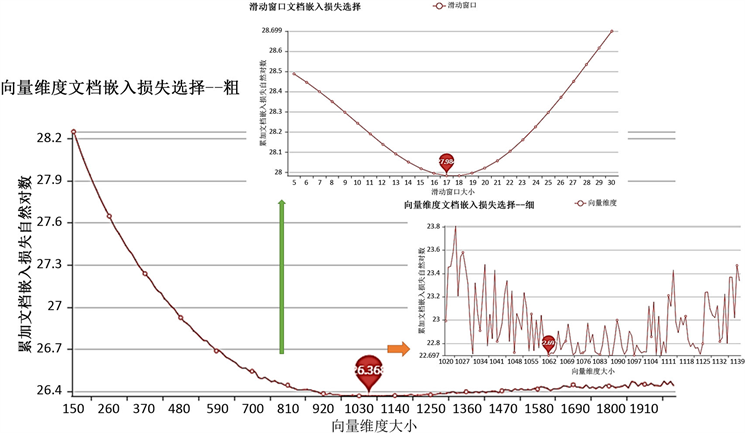Figure 3. Government work report embedding dimension and sliding window selection

4.3. 文档嵌入维度大小设置及其影响

Yin和Shen在其论文中提出了一个理解词嵌入维度的理论框架 。他们定义了PIP损失函数来测量词嵌入之间的差异，通过将词嵌入转化为带噪音的矩阵分解问题，开发了一套基于微扰理论(matrix perturbation theory)的分析技术，发现PIP损失函数有偏差-方差分解(bias-variance decomposition)进而直接证明了词嵌入最佳维度的存在，通过寻找偏差和方差的平衡点可以直接求解理论上最优的维度。

· 深度神经网络对于文本分词序列的过学习，使得文档向量具有较大方差夹杂大量杂音；

· 训练的时间成本和设备成本与文档的规模、维度的大小成正比，越大的文档集和越大的维度设置会带来越长的训练时间和越昂贵的硬件要求。

· 文本越长，最佳嵌入维度越大；

· 文本的单句平均长度越长，滑动窗口越大；

· 滑动窗口通常取文档句子长度的平均词数。

1) 根据待训练文档集的平均长度预估其最佳嵌入维度大小的区间；

2) 在预估区间上根据实际情况设置区间间隔，使用小批量文档集，快速训练，在得到的文档向量上使用通用模型1得到最优维度大小；

3) 绘制文档嵌入损失-嵌入维度图，若所选最优维度处于凹曲线内，决定使用更小的区间间隔再度优化或退出选择；若所选最优维度处于预估区间两端之一或无凹曲线，则应向该方向调整预估区间。

5. 政府工作报告省域可分性

5.1. 省域文档大数据可分性分析

${R}_{1}\left(j,s\right)=\left\{x|{x}^{\left(j\right)}\le s\right\}$,${R}_{2}\left(j,s\right)=\left\{x|{x}^{\left(j\right)}>s\right\}$

XGBoost将许多CART集成联合得到总输出，这种许多使用同一模型、效果不佳的弱分类器，联合在一起使得它们组成一个分类效果超过它们中任一一个弱分类器的强分类器的算法思想为Boosting 。XGBoost支持在特征粒度上并行计算，这使得其对具有高纬度特征的文档向量非常友好，并且其支持列抽样。XGBoost在机器学习领域的天池、Kaggle和DataCastle的比赛中屡获大奖，模型的准确性得到实际检验。不同维度的文档嵌入同胚具有相同数目的相对坐标，对政府工作报告文档大数据进行标签文档训练、预测时，可利用XGBoost算法准确、快速、多线程并行计算的优点，研究不同省份的报告文档是否具有明显的可区分性。

5.2. 省域可分性实验结果与因素分析Figure 4. Provincial separability under different sliding window of government work report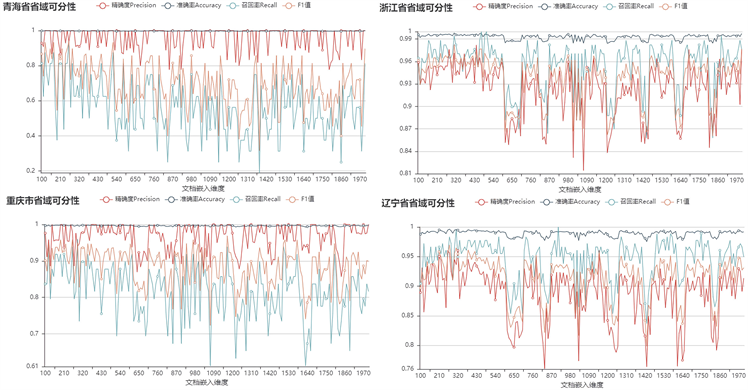Figure 5. Provincial severability in different embedding dimensions of government work reportTable 1. Provincial severability under the optimal dimension of government work report

· 各省省情不一，例如西藏、宁夏等作为少数民族自治区，政府工作侧重民族区域自治，报告内容自然与其他省市不同；

· 各省语言风格不同，为使政府工作报告接地气、入民心，各地官话、晋、湘、赣、吴、闽、粤、客语八大方言  本地特色的语料不同程度地进入到当地的政府工作报告中。

6. 政府工作报告省域相似性

6.1. 省域文档大数据相似性分析

$\text{SPSR}\left(D,{W}_{d}\right)=\frac{\text{SUM}\left(\left\{D={W}_{di}\right\}\right)}{\text{SUM}\left\{{W}_{d}\right\}}$

$\text{SCSR}\left(D,{W}_{d}\right)=\frac{\text{SUM}\left(\left\{D\cong {W}_{di}\right\}\right)}{\text{SUM}\left\{{W}_{d}\right\}}$

$\text{DPSR}\left(D,{W}_{d}\right)=\frac{\text{SUM}\left(\left\{D\ne {W}_{di}\right\}\right)}{\text{SUM}\left\{{W}_{d}\right\}}$

6.2. 省域相似性实验结果与因素分析Table 2. Provincial document similarity in the optimal dimension of government work report

T-SNE是一种可用于高维数据降维的流形学习方法 。文档嵌入向量降维引起的信息损失会随着维数的增加而不能弥补维数优化带来的增益，因此，这里选取200维、训练迭代次数40的文档嵌入向量使用T-SNE降维后得到可视化结果(见图6)。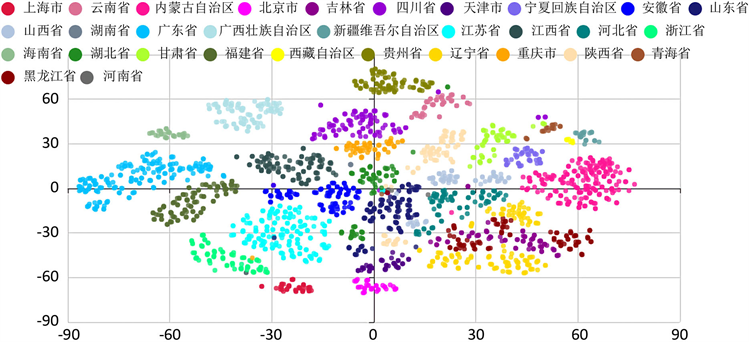Figure 6. Two-dimensional visualization of embedding vectors of government work report after dimensionality reduction by T-SNE

1) 经济活动联系并不是以省市行政区域为分割的，例如长三角经济带，江苏苏州、江苏昆山、浙江嘉兴相对本省省会来说，与上海市经济联系更加紧密，产业配套更加衔接；

2) 社会发展阶段并不是同省市就处于同一水平的，例如江苏无锡与湖南长沙经济总量相差不大，但长沙作为省会城市与同省非省会城市岳阳、衡阳等就不在一个发展阶段上。

1) 以北京市为代表的同地相似率和同市相似率显著高于异省相似率；

2) 以河南省为代表的同地相似率和同市相似率显著低于异省相似率；

3) 以湖北省为代表的同地相似率、同市相似率和异省相似率差别不大。

1) 各地方政府官员的调动频繁程度不一，往往地方主要领导的升迁会相应的伴随着施政方向的变化，政府工作内容和政府工作报告执笔人的变化也相应的反应在各地方政府的同地相似率中；

2) 北京、上海等同地相似率和同市相似率显著高于异省相似率，由于经济地位和政治地位的塔尖位置，其政府的工作重心、行政风格、经济政治体量和卓越的公务员群体都充分渗透到其政府工作报告中显著区别于其他省市，行政风格的创新性和试点试新的领先性体现在政府工作报告的报告内容中，使得它们的政府工作报告表现为同地相似率和同市相似率很高，异省相似率低；

3) 河南和湖南等同地相似率和同市相似率显著低于异省相似率，与其产业链完整度、经济发展水平、地理位置、人口流动、教育资源及民间文化相关，湘楚文化重文教、向往外出闯荡，同时湖南紧邻广东、湖北、重庆，与广州、深圳、重庆、武汉的经济、人口往来密切，河南省内高等教育资源匮乏导致其地方政府的各级政府主要领导在外省市接受高等教育的比例大，同时河南身为人口大省又地处中原，省会城市对省内经济、文化、人口、产业的吸引力不是特别明显；

4) 湖北、四川、陕西同地相似率、同市相似率和异省相似率差别不大，由于武汉、成都、西安分别是中部、西南部、西北部地区的中心城市，其周边省市人口流入带来的文化多样性和省会城市教育、医疗、政治资源的集中性等因素都使得其同地、同时、异省相似率均比较平衡。

7. 结语

Document Embedding Dimension Homeomorphism—Government Work Report Analysis[J]. 计算机科学与应用, 2020, 10(06): 1194-1208. https://doi.org/10.12677/CSA.2020.106124

1. 1. 魏伟, 郭崇慧, 陈静锋. 国务院政府工作报告(1954-2017)文本挖掘及社会变迁研究[J]. 情报学报, 2018, 37(4): 406-421.

2. 2. 何家莉. 覆盖近似空间的连续与同胚映射[J]. 纯粹数学与应用数学, 2019, 35(2): 229-234.

3. 3. Harris, Z. (1954) Distributional Structure. Word, 10, 146-162. https://doi.org/10.1080/00437956.1954.11659520

4. 4. Bengio, Y., Ducharme, R., Vincent, P. and Jauvin, C. (2003) Neural Probabilistic Language Model. Journal of Machine Learning Research, 3, 1137-1155.

5. 5. Yin, Z. and Shen, Y.Y. (2018) On the Dimensionality of Word Embedding. Neural Information Processing Sytems. ComputerScence, Montreal. http://arxiv.org/abs/1812.04224v1

6. 6. Mikolov, T., Sutskever, I., Chen, K., Corrado, G. and Dean, J. (2013) Distributed Representations of Words and Phrases and Their Compositionality. http://arxiv.org/abs/1310.4546v1

7. 7. Le, Q. and Mikolov, T. (2014) Distributed Representations of Sentences and Documents. http://arxiv.org/abs/1405.4053

8. 8. 李欣, 李旸, 王素格. 面向情感聚类的文本相似度计算方法研究[J]. 中文信息学报, 2018, 32(5): 97-104.

9. 9. 朱小飞, 郭嘉丰, 程学旗, 杜攀. 基于流形排序的查询推荐方法[J]. 中文信息学报, 2011, 25(2): 38-43.

10. 10. 罗四维, 赵连伟. 基于谱图理论的流形学习算法[J]. 计算机研究与发展, 2006, 43(7): 1173-1179.

11. 11. Yin. (2018) Understand Functionality and Dimensionality of Vector Embeddings: The Distribu-tional Hypothesis, the Pairwise Innerproduct Loss and Its Bias-Variance Trade-Off. https://arxiv.org/abs/1803.00502

12. 12. 张蕾, 崔勇, 刘静, 江勇, 吴建平. 机器学习在网络空间安全研究中的应用[J]. 计算机学报, 2018, 41(9): 1943-1975.

13. 13. Chen, T. and Guestrin, C. (2016) XGBoost: A Scalable Tree Boosting System. Proceedings of the 22nd ACM SIGKDD International Conference on Knowledge Discovery and Data Mining, August 2016, 785-794. https://doi.org/10.1145/2939672.2939785

14. 14. 徐春华, 刘力. 省域市场潜力、产业结构升级与城乡收入差距——基于空间关联与空间异质性的视角[J]. 农业技术经济, 2015(5): 34-46.

15. 15. 李荣. 官话方言的分区[J]. 方言, 1985(1): 2-5.

16. 16. Maaten, L. and Hinton, G. (2008) Visualizing Data Using t-SNE. Journal of Machine Learning Re-search, 9, 2579-2605.